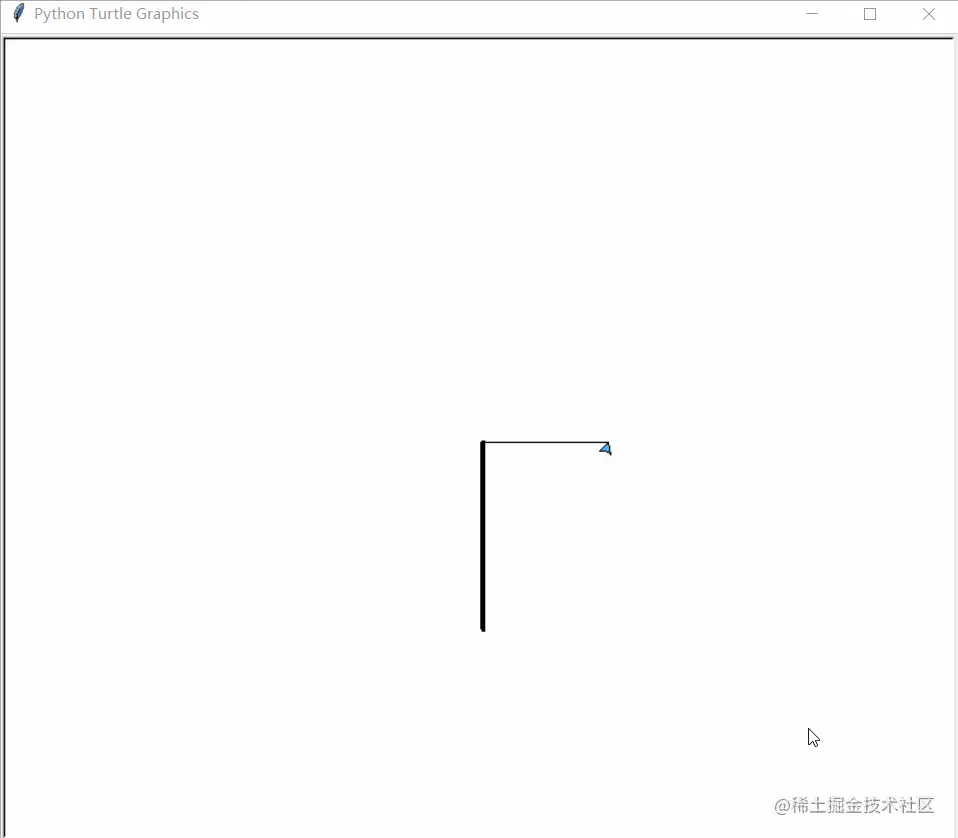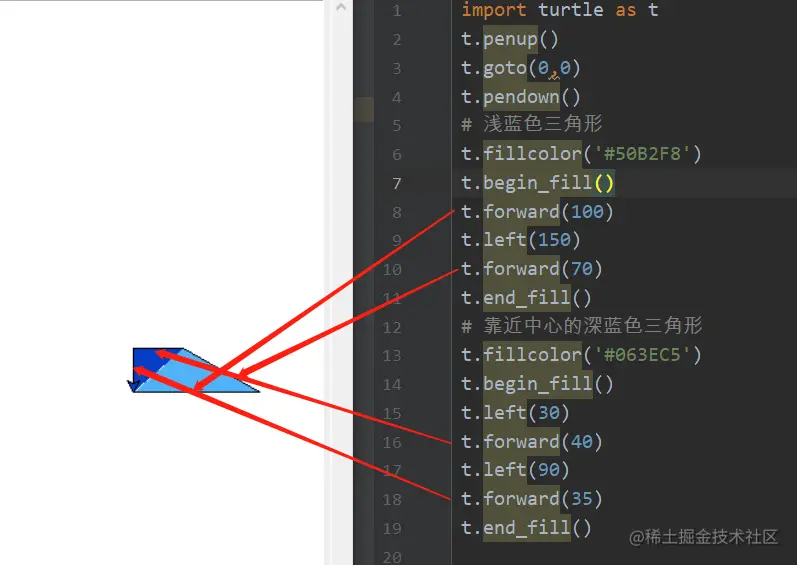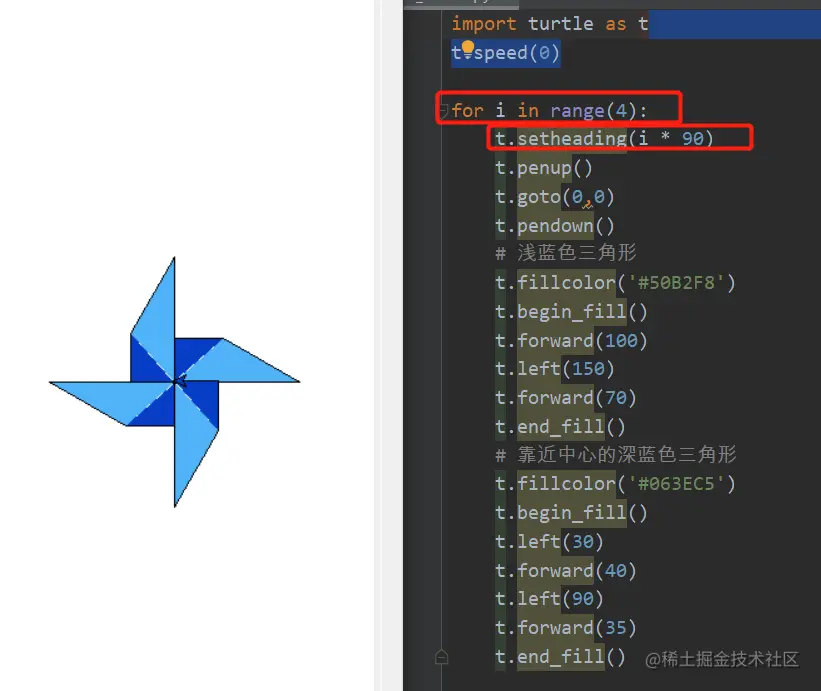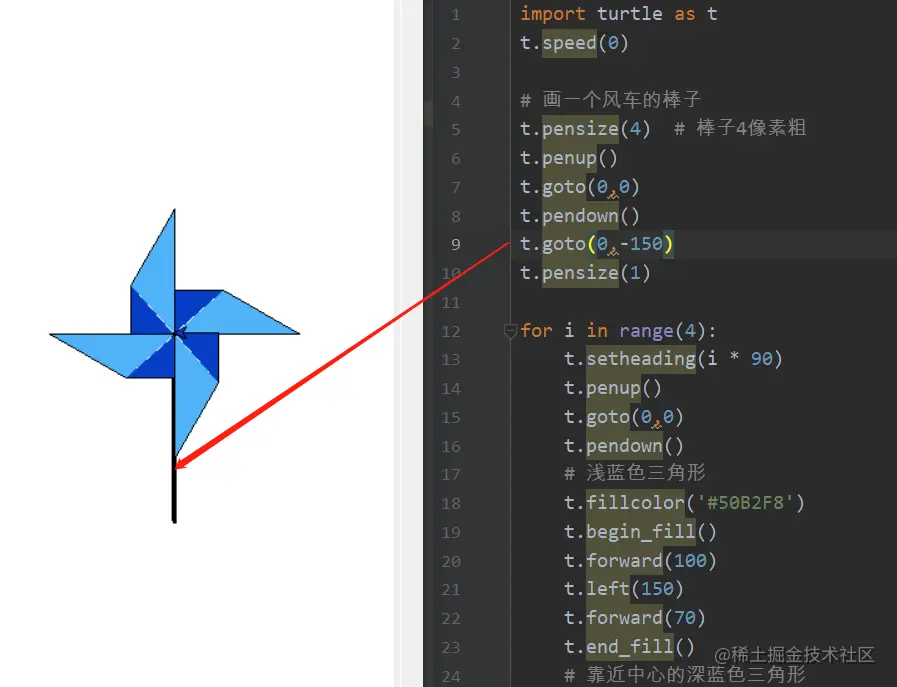# Python draws a spinning windmill with turtle

2022-01-30 07:15:25

Last time I used turtle Made a love , This time, let's make a rotating windmill , First put the picture below , Take a look at the effect after completionNow let's start writing the code

# Design leaves

One leaf can be realized by two triangles, one deep and one shallow , Let's say 0 Take leaves in degrees as an example , The code we write is as follows# Use a loop to generate 4 Leaf

With a leaf , Generate 4 It's easier to have a leaf , We just need to write a loop , Then use... In the loop 90 To specify the lower bearing angle , The rest of the drawing code is the sameAdd a slightly thicker stick under the windmill , We just need to set the thickness of the brush and draw a straight line# The rotation of the windmill

The windmill has been painted , But how can we make it turn ？ See we loop out 4 A leaf code , Where we specified the angle is 90 Multiple , Write the direction of the leaves as fixed , If we change that angle into a variable , You can turn the leaves at different angles . For ease of use , Let's order one first draw function , So that it can receive the angle specified by itself .

Modify the following code

``````import turtle as t
t.speed(0)
def draw(r):
#  Draw a windmill stick
t.pensize(4)  #  bonzi 4 Pixel thick
t.penup()
t.goto(0,0)
t.pendown()
t.goto(0,-150)
t.pensize(1)

for i in range(4):
t.penup()
t.goto(0,0)
t.pendown()
#  Light blue triangle
t.fillcolor('#50B2F8')
t.begin_fill()
t.forward(100)
t.left(150)
t.forward(70)
t.end_fill()
#  Dark blue triangle near the center
t.fillcolor('#063EC5')
t.begin_fill()
t.left(30)
t.forward(40)
t.left(90)
t.forward(35)
t.end_fill()

draw(30)  #  Try different angles , The leaves can turn to the angle we want

t.done()
Copy code ``````

The angle can be changed , But the animation of rotation has not yet , How to do this ？ We can write a loop to call this draw function

``````for r in range(0,360*100,3):  #  Here is the rotation 100 circle , It can also be used. while loop , Keep it spinning , step 3 Corresponding to the speed , It can also be changed to 1、2 Look at the effect
t.tracer(False)
t.clear()
draw(r)
time.sleep(0.01)
t.hideturtle()
t.tracer(True)
Copy code ``````

Okay , Now the windmill can finally turn , Finally, we post the completed code

``````import turtle as t
import time
def draw(r):
#  Draw a windmill stick
t.pensize(4)  #  bonzi 4 Pixel thick
t.penup()
t.goto(0,0)
t.pendown()
t.goto(0,-150)
t.pensize(1)

for i in range(4):
t.penup()
t.goto(0,0)
t.pendown()
#  Light blue triangle
t.fillcolor('#50B2F8')
t.begin_fill()
t.forward(100)
t.left(150)
t.forward(70)
t.end_fill()
#  Dark blue triangle near the center
t.fillcolor('#063EC5')
t.begin_fill()
t.left(30)
t.forward(40)
t.left(90)
t.forward(35)
t.end_fill()

#  With the speed 1 Play the drawing process once
t.speed(1)
draw(0)

for r in range(0,360*100,3):  #  Here is the rotation 100 circle , It can also be used. while loop , Keep it spinning , step 3 Corresponding to the speed , It can also be changed to 1、2 Look at the effect
t.tracer(False)
t.clear()
draw(r)
time.sleep(0.01)
t.hideturtle()
t.tracer(True)

t.done()
Copy code ``````